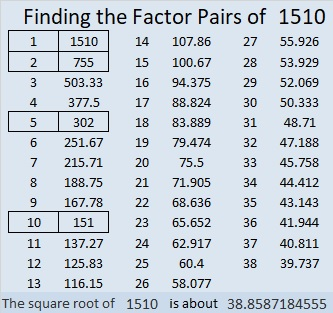# 1510 Challenge Puzzle

### Today’s Puzzle:

Challenge puzzles are like four multiplication tables connected to each other. Use logic to place the factors 1 to 10 in each boldly outlined column or row so that the given clues are the products of the factors you write. I hope you enjoy solving this puzzle as much as I enjoyed making it for you!Here’s an excel file with this week’s puzzles: 10 Factors 1502-1510

### Factors of 1510:

• 1510 is a composite number.
• Prime factorization: 1510 = 2 × 5 × 151.
• 1510 has no exponents greater than 1 in its prime factorization, so √1510 cannot be simplified.
• The exponents in the prime factorization are 1, 1, and 1. Adding one to each exponent and multiplying we get (1 + 1)(1 + 1)(1 + 1) = 2 × 2 × 2 = 8. Therefore 1510 has exactly 8 factors.
• The factors of 1510 are outlined with their factor pair partners in the graphic below.### One More Fact about the Number 1510:

1510 is the hypotenuse of a Pythagorean triple:
906-1208-1510, which is (3-4-5) times 302.